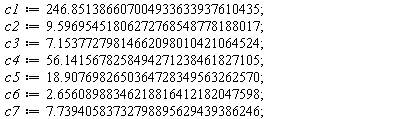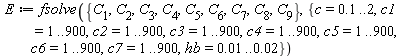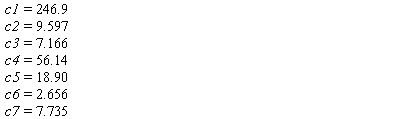## 20 Reputation

10 years, 238 days

## @Carl Love even simpler. Thanks Carl!...

@Carl Love even simpler. Thanks Carl!

## @Kitonum also a neat solution. Thanks!...

@Kitonum also a neat solution. Thanks!

## @Axel Vogt HA! A darn simple solution......

@Axel Vogt HA! A darn simple solution... I guess I had my mind wrapped around some sort of built in functionality... Moments that make you want to crawl underneath a rock. Thanks Axel!

## Clarification...

Acer, Carl, thanks for your comments. Its not that I want a clean slate... Let me clarify and maybe it will make more sense. I might not be using the best approach, but so far it has worked well for me over the last 8 years.

Righ now I am solving Kirchhoff plates and Mindlin plates in one worksheet. Both methods basically use the same variables and I want to compare the results. What I typically do is load all my packages at the begining of my worksheet using the "with" command directly on the worksheet. Once the Kirchhoff plate method is solved it moves onto the Mindlin plate method. However, the variables I am using for Mindlin are no longer variables, but assigned values calculated in the Kirchhoff plate method. (I am using the same variables for ease of programming and comparison, of course I could use a whole different set of variables, but that would defy the point of this question and take a long time to change! Long code!). I thought it might be easy just to unassign the variables and use them again in the Mindlin plate method without having to reload everything else.

I usually hit the restart maple server button and then the execute entire worksheet button anytime I make changes to the code in order to rerun the entire worksheet.

Hope this clears up my reasoning,

Patrick

## Hey John,   Thanks for the proced...

Hey John,

Thanks for the procedure, I have been testing it with only 1 equation. It would appear that the optimization algorithm is having trouble because it is trying to minimize to the smallest possible value and for maple that is the largest negative value it can find. Whereas I am trying to find the values which produce a solution as close to zero as possible regardless of whether or not that solution is positive or negative. Here is my code, suggestions? Also it is inclear to me what "infolevel" does exactly.

infolevel[Optimization] := 4;
4
Digits := 30;
30
a1 := 1.514; a2 := 5.049;
1.514
5.049
Optimization[Minimize](C, {c >= 0, c1 >= 1, c2 >= 1, c3 >= 1, c4 >= 1, c5 >= 1, c6 >= 1, c7 >= 1, hb >= 0.1e-1, c <= 2, c1 <= 900, c2 <= 900, c3 <= 900, c4 <= 900, c5 <= 900, c6 <= 900, c7 <= 900, hb <= 0.2e-1}, iterationlimit = 1000000, initialpoint = [c = 1, hb = 0.1e-1, c1 = 1, c2 = 1, c3 = 1, c4 = 1, c5 = 1, c6 = 1, c7 = 1]);
NLPSolve: calling NLP solver
NLPSolve: using method=sqp
NLPSolve: number of problem variables 9
NLPSolve: number of nonlinear inequality constraints 0
NLPSolve: number of nonlinear equality constraints 0
NLPSolve: number of general linear constraints 18
NLPSolve: feasibility tolerance set to 0.1e-14
NLPSolve: optimality tolerance set to 0.2511886432e-21
NLPSolve: iteration limit set to 1000000
NLPSolve: infinite bound set to 0.10e21
NLPSolve: trying evalf mode
attemptsolution: iterates did not converge, but first-order conditions have been met
attemptsolution: number of major iterations taken 7

[-1.23389380823272547574498378184 10^24  , [
c = 2.00000000000000000000000000000,

c1 = 900.000000000000000000000000000,

c2 = 900.000000000000000000000000000,

c3 = 900.000000000000000000000000000,

c4 = 900.000000000000000000000000000,

c5 = 900.000000000000000000000000000,

c6 = 900.000000000000000000000000000,

c7 = 900.000000000000000000000000000,

hb = 0.0200000000000000000000000000000]]

Thanks,

Pat

## Hey John,   Thanks for the proced...

Hey John,

Thanks for the procedure, I have been testing it with only 1 equation. It would appear that the optimization algorithm is having trouble because it is trying to minimize to the smallest possible value and for maple that is the largest negative value it can find. Whereas I am trying to find the values which produce a solution as close to zero as possible regardless of whether or not that solution is positive or negative. Here is my code, suggestions? Also it is inclear to me what "infolevel" does exactly.

infolevel[Optimization] := 4;
4
Digits := 30;
30
a1 := 1.514; a2 := 5.049;
1.514
5.049
Optimization[Minimize](C, {c >= 0, c1 >= 1, c2 >= 1, c3 >= 1, c4 >= 1, c5 >= 1, c6 >= 1, c7 >= 1, hb >= 0.1e-1, c <= 2, c1 <= 900, c2 <= 900, c3 <= 900, c4 <= 900, c5 <= 900, c6 <= 900, c7 <= 900, hb <= 0.2e-1}, iterationlimit = 1000000, initialpoint = [c = 1, hb = 0.1e-1, c1 = 1, c2 = 1, c3 = 1, c4 = 1, c5 = 1, c6 = 1, c7 = 1]);
NLPSolve: calling NLP solver
NLPSolve: using method=sqp
NLPSolve: number of problem variables 9
NLPSolve: number of nonlinear inequality constraints 0
NLPSolve: number of nonlinear equality constraints 0
NLPSolve: number of general linear constraints 18
NLPSolve: feasibility tolerance set to 0.1e-14
NLPSolve: optimality tolerance set to 0.2511886432e-21
NLPSolve: iteration limit set to 1000000
NLPSolve: infinite bound set to 0.10e21
NLPSolve: trying evalf mode
attemptsolution: iterates did not converge, but first-order conditions have been met
attemptsolution: number of major iterations taken 7

[-1.23389380823272547574498378184 10^24  , [
c = 2.00000000000000000000000000000,

c1 = 900.000000000000000000000000000,

c2 = 900.000000000000000000000000000,

c3 = 900.000000000000000000000000000,

c4 = 900.000000000000000000000000000,

c5 = 900.000000000000000000000000000,

c6 = 900.000000000000000000000000000,

c7 = 900.000000000000000000000000000,

hb = 0.0200000000000000000000000000000]]

Thanks,

Pat

## Lack of procedure knowledge...

Acer, thanks for the reply. However Maple procedures are very new to me and I would like a little clarification. In the first set of code I don't understand what this means within the unapply brackets:

`[indets(Cnew,name)[]] I personnally would have gone along the lines of [c, c1, c2, c3, c4, c5, c6, c7, hb].Also, for the second set of code, I have no idea what is going on here:`
```Cproc(1.0,
246.851, 9.597, 7.153, 56.14, 18.91, 2.656, 7.73,
0.012);

11
-0.9931016321 10

f(1.0,
246.851, 9.597, 7.153, 56.14, 18.91, 2.656, 7.73,
0.012);

-0.992357302391520534067512587085549339330928664664135982173091890590934784\

11
6556567851795358311687275614 10Thanks!Pat```
` `

## Lack of procedure knowledge...

Acer, thanks for the reply. However Maple procedures are very new to me and I would like a little clarification. In the first set of code I don't understand what this means within the unapply brackets:

`[indets(Cnew,name)[]] I personnally would have gone along the lines of [c, c1, c2, c3, c4, c5, c6, c7, hb].Also, for the second set of code, I have no idea what is going on here:`
```Cproc(1.0,
246.851, 9.597, 7.153, 56.14, 18.91, 2.656, 7.73,
0.012);

11
-0.9931016321 10

f(1.0,
246.851, 9.597, 7.153, 56.14, 18.91, 2.656, 7.73,
0.012);

-0.992357302391520534067512587085549339330928664664135982173091890590934784\

11
6556567851795358311687275614 10Thanks!Pat```
` `

## Trouble with codegen...

Hi Carl, I appreciate you helping out a lot. To answer your question, even with the uncompressed set of equations, fsolve returned unevaluated rather quickly which is what prompted my original question. At this point it is clear that fsolve will not resolve at the current level of precision. However, I guess that is not my final goal either as per my new question.

I've been trying to implement your procedure above, but it seems that my lack of maple procedures knowledge is hindering me. It seems after I run the optimize function I get back the exact same set of equations I had earlier. If you could direct my ingorance that would be great. Notice that certain equations give solutions to only 1/10 decimal order. I could create new equations with more digits which would also be solved with a1, a2, c, hb and c1..c7 containing more significant digits which would produce solutions of much smaller magnitude. However that isn't really the point:

Digits := 30;
30
G := unapply(C, [c, c1, c2, c3, c4, c5, c6, c7, hb, a1, a2]);
[Length of output exceeds limit of 1000000]

with(codegen):

Q := optimize(G, 'tryhard');
[Length of output exceeds limit of 1000000]
R := apply(Q, c, c1, c2, c3, c4, c5, c6, c7, hb, a1, a2);
[Length of output exceeds limit of 1000000]

a1 := 1.51401039732306275167060829561;
1.51401039732306275167060829561
a2 := 5.04882865932273295537727396226;
5.04882865932273295537727396226

c := 1;
1
hb := 0.012;
0.012
c1 := 246.851386607004933633937610435;
c2 := 9.59695451806272768548778188017;
c3 := 7.15377279814662098010421064524;
c4 := 56.1415678258494271238461827105;
c5 := 18.9076982650364728349563262570;
c6 := 2.65608988346218816412182047598;
c7 := 7.73940583732798895629439386246;
246.851386607004933633937610435
9.59695451806272768548778188017
7.15377279814662098010421064524
56.1415678258494271238461827105
18.9076982650364728349563262570
2.65608988346218816412182047598
7.73940583732798895629439386246
R;
[[-.906584860938465e-5],[.9816362000e-13],[.14327551436416154162],[-.1369438000e-13],[-.1288000000e-16],[-.2980383418e-10],[-.514339377242618e-5],[.2044636200e-12],[.15920025295044888362]]

## Trouble with codegen...

Hi Carl, I appreciate you helping out a lot. To answer your question, even with the uncompressed set of equations, fsolve returned unevaluated rather quickly which is what prompted my original question. At this point it is clear that fsolve will not resolve at the current level of precision. However, I guess that is not my final goal either as per my new question.

I've been trying to implement your procedure above, but it seems that my lack of maple procedures knowledge is hindering me. It seems after I run the optimize function I get back the exact same set of equations I had earlier. If you could direct my ingorance that would be great. Notice that certain equations give solutions to only 1/10 decimal order. I could create new equations with more digits which would also be solved with a1, a2, c, hb and c1..c7 containing more significant digits which would produce solutions of much smaller magnitude. However that isn't really the point:

Digits := 30;
30
G := unapply(C, [c, c1, c2, c3, c4, c5, c6, c7, hb, a1, a2]);
[Length of output exceeds limit of 1000000]

with(codegen):

Q := optimize(G, 'tryhard');
[Length of output exceeds limit of 1000000]
R := apply(Q, c, c1, c2, c3, c4, c5, c6, c7, hb, a1, a2);
[Length of output exceeds limit of 1000000]

a1 := 1.51401039732306275167060829561;
1.51401039732306275167060829561
a2 := 5.04882865932273295537727396226;
5.04882865932273295537727396226

c := 1;
1
hb := 0.012;
0.012
c1 := 246.851386607004933633937610435;
c2 := 9.59695451806272768548778188017;
c3 := 7.15377279814662098010421064524;
c4 := 56.1415678258494271238461827105;
c5 := 18.9076982650364728349563262570;
c6 := 2.65608988346218816412182047598;
c7 := 7.73940583732798895629439386246;
246.851386607004933633937610435
9.59695451806272768548778188017
7.15377279814662098010421064524
56.1415678258494271238461827105
18.9076982650364728349563262570
2.65608988346218816412182047598
7.73940583732798895629439386246
R;
[[-.906584860938465e-5],[.9816362000e-13],[.14327551436416154162],[-.1369438000e-13],[-.1288000000e-16],[-.2980383418e-10],[-.514339377242618e-5],[.2044636200e-12],[.15920025295044888362]]

## What I am trying to do...

Ok I've been playing around with my code a lot, without actually trying the suggestions yet. However I really would like you guys to understand what I am trying to do.

So I have created a new .m file with the equations with two extra variables (a1 and a2). What I had previously done was set a1 and a2 beforehand. The file itself is here:

Cvector_with_a.txt  (once again rename the .txt file extension to .m)

Setting the digits to 30, a1:=1.51401039732306275167060829561 and a2:=5.04882865932273295537727396226, then a known solution to the 9 equations and 9 unknowns within reason is:

c=1, hb=0.012 and any combination of c1..c7 such that:Since a1 and a2 are collected experimentally, they are only accurate to about 4 digits. Therefore I would like to get a solution similar to the one above accurate to about 4 digits, by setting a1:=1.514 and a2:=5.049. The problem is that these equations seem to be highly sensitive to numerical errors due to the 9th order polynomial equations. If you reduce to a1:=1.514 and a2:=5.049 then the above values are off by a factor of 10^5.

Any suggestions?

Thanks again for all your help, it is really appreciated.

Pat

## What I am trying to do...

Ok I've been playing around with my code a lot, without actually trying the suggestions yet. However I really would like you guys to understand what I am trying to do.

So I have created a new .m file with the equations with two extra variables (a1 and a2). What I had previously done was set a1 and a2 beforehand. The file itself is here:

Cvector_with_a.txt    (once again rename the .txt file extension to .m)

Setting the digits to 30, a1:=1.51401039732306275167060829561 and a2:=5.04882865932273295537727396226, then a known solution to the 9 equations and 9 unknowns within reason is:

c=1, hb=0.012 and any combination of c1..c7 such that:Since a1 and a2 are collected experimentally, they are only accurate to about 4 digits. Therefore I would like to get a solution similar to the one above accurate to about 4 digits, by setting a1:=1.514 and a2:=5.049. The problem is that these equations seem to be highly sensitive to numerical errors due to the 9th order polynomial equations. If you reduce to a1:=1.514 and a2:=5.049 then the above values are off by a factor of 10^5.

Any suggestions?

Thanks again for all your help, it's really appreciated.

Pat

## What I am trying to do...

Ok I've been playing around with my code a lot, without actually trying the suggestions yet. However I really would like you guys to understand what I am trying to do.

So I have created a new .m file with the equations with two extra variables (a1 and a2). What I had previously done was set a1 and a2 beforehand. The file itself is here:

Cvector_with_a.txt    (once again rename the .txt file extension to .m)

Setting the digits to 30, a1:=1.51401039732306275167060829561 and a2:=5.04882865932273295537727396226, then a known solution to the 9 equations and 9 unknowns within reason is:

c=1, hb=0.012 and any combination of c1..c7 such that:Since a1 and a2 are collected experimentally, they are only accurate to about 4 digits. Therefore I would like to get a solution similar to the one above accurate to about 4 digits, by setting a1:=1.514 and a2:=5.049. The problem is that these equations seem to be highly sensitive to numerical errors due to the 9th order polynomial equations. If you reduce to a1:=1.514 and a2:=5.049 then the above values are off by a factor of 10^5.

Any suggestions?

Thanks again for all your help, it's really appreciated.

Pat

## .m files...

Thanks for all the help guys you are giving me lots of new avenues to explore. Ok so here is the .m files I have the same equations created using either 4 digits or 15 digits, your choice on what you feel is the minimum amount of digits you need (Please change the file extension from .txt to .m since this forum does not seem to allow the upload of .m files):

Cvector_equations_4d.txt

Cvector_equations_15.txt

The files themselves are just a 9x1 C vector where every entry in C is an equation as per the notation I used in fsolve:The expected solution is c = 1, hb = 0.012 andAccuracy to only about 4 digits is sufficient.

Since we are solving 9 equations with 9 unkowns but the polynomial equations are 9th order, we expect many possible solutions. This is why we set the ranges for the values of the unkowns in fsolve. the values of c1..c7 do not need to be ordered as per described above. Any value in the vector can be associated to any value of c1..c7.

Pat

PS It is getting late and I realise now that simplifications made earlier in my code may be causing large numerical errors. I will post a description of this tomorrow along with more .m files which will allow you to understand what I am attempting to do more clearly.

## .m files...

Thanks for all the help guys you are giving me lots of new avenues to explore. Ok so here is the .m files I have the same equations created using either 4 digits or 15 digits, your choice on what you feel is the minimum amount of digits you need (Please change the file extension from .txt to .m since this forum does not seem to allow the upload of .m files):

Cvector_equations_4d.txt

Cvector_equations_15.txt

The files themselves are just a 9x1 C vector where every entry in C is an equation as per the notation I used in fsolve:The expected solution is c = 1, hb = 0.012 andAccuracy to only about 4 digits is sufficient.

Since we are solving 9 equations with 9 unkowns but the polynomial equations are 9th order, we expect many possible solutions. This is why we set the ranges for the values of the unkowns in fsolve. the values of c1..c7 do not need to be ordered as per described above. Any value in the vector can be associated to any value of c1..c7.

Pat

PS It is getting late and I realise now that simplifications made earlier in my code may be causing large numerical errors. I will post a description of this tomorrow along with more .m files which will allow you to understand what I am attempting to do more clearly.

 1 2Page 1 of 2
﻿